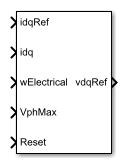# PMSM Current Controller with Pre-Control

Discrete-time permanent magnet synchronous machine current controller with pre-control

•Libraries:
Simscape / Electrical / Control / PMSM Control

## Description

The PMSM Current Controller with Pre-Control block implements a discrete-time PI-based permanent magnet synchronous machine (PMSM) current controller in the rotor d-q reference frame with internal feedforward pre-control.

You typically use this block in a series of blocks making up a control structure.

• You can generate a current reference in the d-q frame to be used as an input to this block with a PMSM Current Reference Generator.

• You can obtain a voltage reference in the abc domain by converting the output of this block using an Inverse Park Transform block.

You can see an example of a full control structure, from machine measurements to machine inputs, in the PMSM Field-Oriented Control block.

### Equations

The block is discretized using the backward Euler method due to its first-order simplicity and its stability.

Two PI current controllers implemented in the rotor reference frame produce the reference voltage vector:

`${v}_{d}^{ref}=\left({K}_{p_id}+{K}_{i_id}\frac{{T}_{s}z}{z-1}\right)\left({i}_{d}^{ref}-{i}_{d}\right)+{v}_{d_FF},$`

and

`${v}_{q}^{ref}=\left({K}_{p_iq}+{K}_{i_iq}\frac{{T}_{s}z}{z-1}\right)\left({i}_{q}^{ref}-{i}_{q}\right)+{v}_{q_FF},$`

where:

• ${v}_{d}^{ref}$ and ${v}_{q}^{ref}$ are the d-axis and q-axis reference voltages, respectively.

• ${i}_{d}^{ref}$ and ${i}_{q}^{ref}$ are the d-axis and q-axis reference currents, respectively.

• ${i}_{d}$ and ${i}_{q}$ are the d-axis and q-axis currents, respectively.

• Kp_id and Kp_iq are the proportional gains for the d-axis and q-axis controllers, respectively.

• Ki_id and Ki_iq are the integral gains for the d-axis and q-axis controllers, respectively.

• Ts is the sample time of the discrete controller.

• vd_FF and vq_FF are the feedforward voltages for the d-axis and q-axis, respectively.

The feedforward voltages are obtained from the machine mathematical equations:

`${v}_{d_FF}=-{\omega }_{e}{L}_{q}{i}_{q}^{ref},$`

and

`${v}_{q_FF}={\omega }_{e}\left({L}_{d}{i}_{d}^{ref}+{\psi }_{m}\right),$`

where:

• ωe is the rotor electrical velocity.

• Ld and Lq are the d-axis and q-axis inductances, respectively.

• ψm is the permanent magnet flux linkage.

### Zero Cancellation

Using PI control results in a zero in the closed-loop transfer function, which can result in undesired overshoot in the closed-loop response. This zero can be canceled by introducing a zero-cancelation block in the feedforward path. The zero cancellation transfer functions in discrete time are:

`${G}_{ZC_id}\left(z\right)=\frac{\frac{{T}_{s}{K}_{i_id}}{{K}_{p_id}}}{z+\left(\frac{{T}_{s}-\frac{{K}_{p_id}}{{K}_{i_id}}}{\frac{{K}_{p_id}}{{K}_{i_id}}}\right)},$`

and

`${G}_{ZC_iq}\left(z\right)=\frac{\frac{{T}_{s}{K}_{i_iq}}{{K}_{p_iq}}}{z+\left(\frac{{T}_{s}-\frac{{K}_{p_iq}}{{K}_{i_iq}}}{\frac{{K}_{p_iq}}{{K}_{i_iq}}}\right)}.$`

### Voltage Saturation

Saturation must be imposed when the stator voltage vector exceeds the voltage phase limit Vph_max:

`$\sqrt{{v}_{d}^{2}+{v}_{q}^{2}}\le {V}_{ph_max},$`

where vd and vq are the d-axis and q-axis voltages, respectively.

In the case of axis prioritization, the voltages v1 and v2 are introduced, where:

• v1 = vd and v2 = vq for d-axis prioritization.

• v1 = vq and v2 = vd for q-axis prioritization.

The constrained (saturated) voltages ${v}_{1}^{sat}$ and ${v}_{2}^{sat}$ are obtained as follows:

`${v}_{1}^{sat}=\text{min}\left(\mathrm{max}\left({v}_{1}^{unsat},-{V}_{ph_max}\right),{V}_{ph_max}\right)$`

and

`${v}_{2}^{sat}=\text{min}\left(\mathrm{max}\left({v}_{2}^{unsat},-{V}_{2_max}\right),{V}_{2_max}\right),$`

where:

• ${v}_{1}^{unsat}$ and ${v}_{2}^{unsat}$ are the unconstrained (unsaturated) voltages.

• v2_max is the maximum value of v2 that does not exceed the voltage phase limit, given by ${v}_{2_max}=\sqrt{{\left({V}_{ph_max}\right)}^{2}-{\left({v}_{1}^{sat}\right)}^{2}}.$

In the case that the direct and quadrature axes have the same priority (d-q equivalence), the constrained voltages are obtained as follows:

`${v}_{d}^{sat}=\text{min}\left(\mathrm{max}\left({v}_{d}^{unsat},-{V}_{d_max}\right),{V}_{d_max}\right)$`

and

`${v}_{q}^{sat}=\text{min}\left(\mathrm{max}\left({v}_{q}^{unsat},-{V}_{q_max}\right),{V}_{q_max}\right),$`

where:

`${V}_{d_max}=\frac{{V}_{ph_max}|{v}_{d}^{unsat}|}{\sqrt{{\left({v}_{d}^{unsat}\right)}^{2}+{\left({v}_{q}^{unsat}\right)}^{2}}}$`

and

`${V}_{q_max}=\frac{{V}_{ph_max}|{v}_{q}^{unsat}|}{\sqrt{{\left({v}_{d}^{unsat}\right)}^{2}+{\left({v}_{q}^{unsat}\right)}^{2}}}.$`

### Integral Anti-Windup

An anti-windup mechanism is employed to avoid saturation of integrator output. In such a situation, the integrator gains become:

`${K}_{i_id}+{K}_{aw_id}\left({v}_{d}^{sat}-{v}_{d}^{unsat}\right)$`

and

`${K}_{i_iq}+{K}_{aw_iq}\left({v}_{q}^{sat}-{v}_{q}^{unsat}\right),$`

where Kaw_id, Kaw_iq, and Kaw_if are the anti-windup gains for the d-axis, q-axis, and field controllers, respectively.

### Assumptions

• The plant model for direct and quadrature axis can be approximated with a first-order system.

• This control solution is used only for permanent magnet synchronous motors with sinusoidal flux distribution and field windings.

## Ports

### Input

expand all

Desired d- and q-axis currents for control of a PMSM, in A.

Data Types: `single` | `double`

Actual d- and q-axis currents of the controlled PMSM, in A.

Data Types: `single` | `double`

Rotor electrical velocity used for feedforward pre-control, in rad/s.

Data Types: `single` | `double`

Maximum allowable voltage in each phase, in V.

Data Types: `single` | `double`

External reset signal (rising edge) for integrators.

Data Types: `single` | `double`

### Output

expand all

Desired d- and q-axis voltages for control of a PMSM, in V.

Data Types: `single` | `double`

## Parameters

expand all

Control Parameters

Proportional gain of the PI controller used for direct-axis current control.

Integrator gain of the PI controller used for direct-axis current control.

Anti-windup gain of the PI controller used for direct-axis current control.

Proportional gain of the PI controller used for quadrature-axis current control.

Integrator gain of the PI controller used for quadrature-axis current control.

Anti-windup gain of the PI controller used for quadrature-axis current control.

Sample time for the block (-1 for inherited). If you use this block inside a triggered subsystem, set the sample time to -1. If you use this block in a continuous variable-step model, you can specify the sample time explicitly.

Prioritize or maintain the ratio between d- and q-axes when the block limits voltage.

Enable or disable zero-cancellation on the feedforward path.

Enable or disable pre-control voltage.

Pre-Control Parameters

Direct-axis current vector used in the lookup tables for parameters determination. For constant machine parameters, do not change the default.

Quadrature-axis current vector used in the lookup tables used to determine parameters. For constant machine parameters, do not change the default.

Ld matrix used as lookup-table data. For constant machine parameters change only the constant factor, for example, Ld * ```ones(3, 3)```.

Lq matrix used as lookup-table data. For constant machine parameters change only the constant factor, e.g., Lq * ```ones(3, 3)```.

Permanent magnet flux linkage matrix used in the lookup table. For constant machine parameters change only the constant factor, for example psim * `ones(3, 3)`.

 Bernardes, T., V. F. Montagner, H. A. Gründling, and H. Pinheiro. "Discrete-time sliding mode observer for sensorless vector control of permanent magnet synchronous machine." IEEE Transactions on Industrial Electronics. Vol. 61, Number 4, 2014, pp. 1679–1691.

 Carpiuc, S., and C. Lazar. "Fast real-time constrained predictive current control in permanent magnet synchronous machine-based automotive traction drives." IEEE Transactions on Transportation Electrification. Vol.1, Number 4, 2015, pp. 326–335.